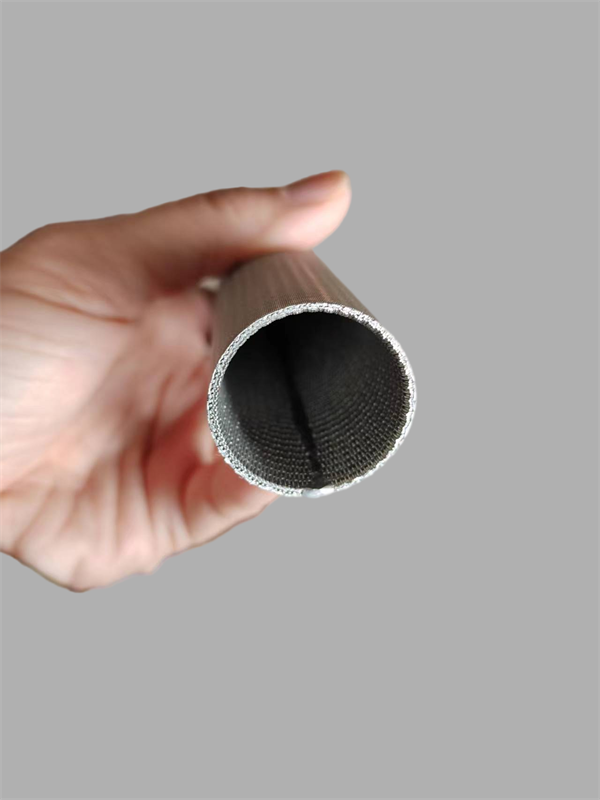FILTALLOY

TECHNOLOGY LTD.

Metal Filter Elements & Materials

# How to Calculate The Differential Pressure of Sintered Wire Mesh FilterThe differential pressure of a sintered wire mesh filter can be calculated using the following formula:

ΔP = (K × μ × L × Q^2) / (d^4 × ρ)

• ΔP = Differential pressure in Pascal (Pa)
• K = Resistance coefficient of the filter
• μ = Dynamic viscosity of the fluid in Pascal-second (Pa·s)
• L = Length of the filter in meters (m)
• Q = Volume flow rate of the fluid in cubic meters per second (m^3/s)
• d = Diameter of the wire in meters (m)
• ρ = Density of the fluid in kilograms per cubic meter (kg/m^3)

Note: The resistance coefficient (K) can be obtained from the manufacturer’s data sheet or by conducting a pressure drop test using a reference fluid. The dynamic viscosity (μ) and density (ρ) of the fluid can be obtained from the fluid properties table or by conducting a viscosity and density test. The length (L), volume flow rate (Q), and diameter (d) of the filter can be measured experimentally.

## More To Explore

### What is Stainless Steel

Stainless Steel Introduction   Stainless steel is a versatile and popular material used in a variety of industries due to its numerous beneficial properties. This

### Where is The Sintered Mesh Filter Cartridge Used?

Sintered mesh filter cartridges Sintered mesh filter cartridges are designed to filter liquids or gases in high-temperature environments, as well as to withstand high and

### Why Choose Filtalloy On SPF Filter Disc

SPL/DPL Filter Disc is a highly effective filtration system that is designed to effectively remove impurities and contaminants from various types of oils. As a